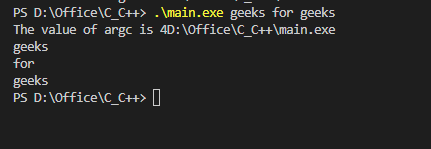# main Function in C

The main function is an integral part of the programming languages such as C, C++, and Java. The main function in C is the entry point of a program where the execution of a program starts. It is a user-defined function that is mandatory for the execution of a program because when a C program is executed, the operating system starts executing the statements in the main() function.

## Syntax of C main() Function

```return_type main() {
// Statement 1;
// Statement 2;
// and so on..
return;
}```

We can write the main function in many ways in C language as follows:

`int main(){} or int main(void){}`
`main(){} or void main(){} or main(void){} or void main(void){}`

In the above notations, int means integer return type, and void return type means that does not return any information, or (void) or () means that does not take any information.

## Important Points about C main Function

• It is the function where the program’s execution starts.
• Every program has exactly one main function.
• The name of this function should be “main” not anything else.
• The main function always returns an integer value or void.
• The main function is called by OS, not the user.

## Types of C main Functions

1. Main function with no arguments and void return type
2. Main function with no arguments and int return type
3. Main function with the Command Line Arguments

### 1. Main Function with No Arguments and Void Return Type

Let’s see the example of the main function inside which we have written a simple program for printing a string. In the below code, first, we include a header file and then we define a main function inside which we write a statement to print a string using printf() function. The main function is called by the operating system itself and then executes all statements inside this function.

Syntax

```void main()
{
// Function body
}```

The above function is equivalent to:

```void main (void)
{
// Function Body
}```

Example

## C

 `// C Program to show main with no return type and no` `// arguments` `#include `   `// Defining a main function` `void` `main()` `{`   `    ``// code` `    ``printf``(``"Hello Geek!"``);` `}`

Output

`Hello Geek!`

Note: The return type of main function according to C standard should be int only. Even if your compiler is able to run void main(), it is recommended to avoid it.

### 2. Main Function with No Arguments and int Return Type

In this example, we use the int return type in the main() function that indicates the exit status of the program. The exit status is a way for the program to communicate to the operating system whether the program was executed successfully or not. The convention is that a return value of 0 indicates that the program was completed successfully, while any other value indicates that an error occurred.

Syntax

```int main()
{
// Function body
}```

or

```int main(void)
{
// Function Body
}```

Example

## C

 `// C Program to show the main function with int return type` `// and no arguments` `#include `   `int` `main()` `{` `    ``printf``(``"Hello Geek!"``);` `    ``return` `0;` `}`

Output

`Hello Geek!`

### 3. Main Function with the Command Line Arguments

In this example, we have passed some arguments in the main() function as seen in the below code. These arguments are called command line arguments and these are given at the time of executing a program.

The first argument argc means argument count which means it stores the number of arguments passed in the command line and by default, its value is 1 when no argument is passed.

The second argument is a char pointer array argv[] which stores all the command line arguments passed. We can also see in the output when we run the program without passing any command line argument the value of argc is 1.

Syntax

```int main(int argc, char* argv[])
{
// Function body
}```

Example

## C

 `// C Program to illustrate the main function with command line arguments` `#include `   `int` `main(``int` `argc, ``char``* argv)` `{`   `    ``// printing the coundt of arguments` `    ``printf``(``"The value of argc is %d\n"``, argc);` `    ``// prining each argument` `    ``for` `(``int` `i = 0; i < argc; i++) {` `        ``printf``(``"%s \n"``, argv[i]);` `    ``}`   `    ``return` `0;` `}`

Output

```The value of argc is 1